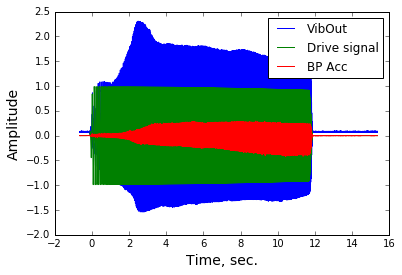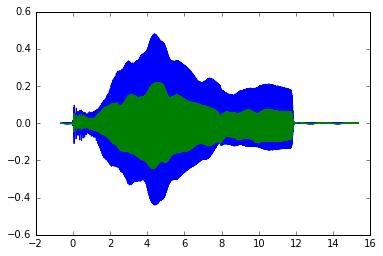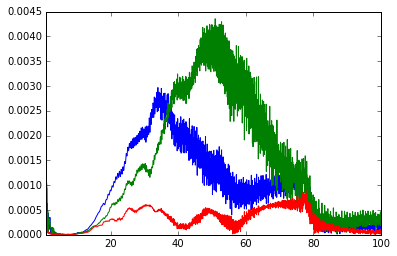# MatLab_Apply_Geo_FFT

by Farnyuh Menq

NHERI@UTexas site Manager

2017/10/25

This notebook open a MatLab data file, apply geophone calibrtion factor and FFT.

In :
# import all the libraries needed
%matplotlib inline
import matplotlib.pyplot as plt
import scipy.io as sio
import numpy as np
import math
import os


# Step 1: Read channel map and calibration factors from an csv file.

In :
#folder = r'C:\Users\fymenq.CAEE-MENQNHERI2\Desktop\201710_Sinkhole'
#folder = folder + '\\'
filename =  'Sinkhole_cal_ChMap.csv'
print (Cal_Fac)

[[   1.    225.      0.      0.     12.      1.       nan    4.81    2.06
0.68    1.      1.  ]
[   2.    235.      0.      0.     11.      1.       nan    4.81    2.06
0.68    1.      1.  ]
[   3.    245.      0.      0.     10.      1.       nan    4.81    2.06
0.68    1.      1.  ]
[   4.    255.      0.      0.      9.      1.       nan    4.81    2.06
0.68    1.      1.  ]
[   5.    265.      0.      0.      8.      1.       nan    4.81    2.06
0.68    1.      1.  ]
[   6.    275.      0.      0.      7.      1.       nan    4.81    2.06
0.68    1.      1.  ]
[   7.    285.      0.      0.      6.      1.       nan    4.81    2.06
0.68    1.      1.  ]
[   8.    295.      0.      0.      5.      1.       nan    4.81    2.06
0.68    1.      1.  ]
[   9.    305.      0.      0.      4.      1.       nan    4.81    2.06
0.68    1.      1.  ]
[  10.    315.      0.      0.      3.      1.       nan    4.81    2.06
0.68    1.      1.  ]
[  11.    325.      0.      0.      2.      1.       nan    4.81    2.06
0.68    1.      1.  ]
[  12.    335.      0.      0.      1.      1.       nan    4.81    2.06
0.68    1.      1.  ]
[  13.    225.     10.      0.     13.      1.       nan    4.81    2.06
0.68    1.      1.  ]
[  14.    235.     10.      0.     14.      1.       nan    4.81    2.06
0.68    1.      1.  ]
[  15.    245.     10.      0.     15.      1.       nan    4.81    2.06
0.68    1.      1.  ]
[  16.    255.     10.      0.     16.      1.       nan    4.81    2.06
0.68    1.      1.  ]
[  17.    265.     10.      0.     17.      1.       nan    4.81    2.06
0.68    1.      1.  ]
[  18.    275.     10.      0.     18.      1.       nan    4.81    2.06
0.68    1.      1.  ]
[  19.    285.     10.      0.     19.      1.       nan    4.81    2.06
0.68    1.      1.  ]
[  20.    295.     10.      0.     20.      1.       nan    4.81    2.06
0.68    1.      1.  ]
[  21.    305.     10.      0.     21.      1.       nan    4.81    2.06
0.68    1.      1.  ]
[  22.    315.     10.      0.     22.      1.       nan    4.81    2.06
0.68    1.      1.  ]
[  23.    325.     10.      0.     23.      1.       nan    4.81    2.06
0.68    1.      1.  ]
[  24.    335.     10.      0.     24.      1.       nan    4.81    2.06
0.68    1.      1.  ]
[  25.    225.     20.      0.     36.      2.       nan    4.81    2.06
0.68    1.      1.  ]
[  26.    235.     20.      0.     35.      2.       nan    4.81    2.06
0.68    1.      1.  ]
[  27.    245.     20.      0.     34.      2.       nan    4.81    2.06
0.68    1.      1.  ]
[  28.    255.     20.      0.     33.      2.       nan    4.81    2.06
0.68    1.      1.  ]
[  29.    265.     20.      0.     32.      2.       nan    4.81    2.06
0.68    1.      1.  ]
[  30.    275.     20.      0.     31.      2.       nan    4.81    2.06
0.68    1.      1.  ]
[  31.    285.     20.      0.     30.      2.       nan    4.81    2.06
0.68    1.      1.  ]
[  32.    295.     20.      0.     29.      2.       nan    4.81    2.06
0.68    1.      1.  ]
[  33.    305.     20.      0.     28.      2.       nan    4.81    2.06
0.68    1.      1.  ]
[  34.    315.     20.      0.     27.      2.       nan    4.81    2.06
0.68    1.      1.  ]
[  35.    325.     20.      0.     26.      2.       nan    4.81    2.06
0.68    1.      1.  ]
[  36.    335.     20.      0.     25.      2.       nan    4.81    2.06
0.68    1.      1.  ]
[  37.    225.     30.      0.     37.      2.       nan    4.81    2.06
0.68    1.      1.  ]
[  38.    235.     30.      0.     38.      2.       nan    4.81    2.06
0.68    1.      1.  ]
[  39.    245.     30.      0.     39.      2.       nan    4.81    2.06
0.68    1.      1.  ]
[  40.    255.     30.      0.     40.      2.       nan    4.81    2.06
0.68    1.      1.  ]
[  41.    265.     30.      0.     41.      2.       nan    4.81    2.06
0.68    1.      1.  ]
[  42.    275.     30.      0.     42.      2.       nan    4.81    2.06
0.68    1.      1.  ]
[  43.    285.     30.      0.     43.      2.       nan    4.81    2.06
0.68    1.      1.  ]
[  44.    295.     30.      0.     44.      2.       nan    4.81    2.06
0.68    1.      1.  ]
[  45.    305.     30.      0.     45.      2.       nan    4.81    2.06
0.68    1.      1.  ]
[  46.    315.     30.      0.     46.      2.       nan    4.81    2.06
0.68    1.      1.  ]
[  47.    325.     30.      0.     47.      2.       nan    4.81    2.06
0.68    1.      1.  ]
[  48.    335.     30.      0.     48.      2.       nan    4.81    2.06
0.68    1.      1.  ]
[    nan     nan     nan     nan     nan     nan     nan     nan     nan
nan     nan     nan]
[    nan     nan     nan     nan     nan     nan     nan     nan     nan
nan     nan     nan]
[    nan     nan     nan     nan     nan     nan     nan     nan     nan
nan     nan     nan]
[    nan     nan     nan     nan     nan     nan     nan     nan     nan
nan     nan     nan]
[    nan     nan     nan     nan     nan     nan     nan     nan     nan
nan     nan     nan]
[    nan     nan     nan     nan     nan     nan     nan     nan     nan
nan     nan     nan]]


# Step 2: Open the MatLab data file

In :
folder = r'C:\Users\fymenq.CAEE-MENQNHERI2\Desktop\Static\201710_Sinkhole\20171024\20171024 Sinkhole test.syn\MATLAB'
folder = folder + '\\'

MatLab_filename =  'DPsv00040.mat' # p-mode
# Read seg2 file using the obspy library
plt.plot (my_data['X50'][:,0], my_data['X50'][:,1],
my_data['X49'][:,0], my_data['X49'][:,1],
my_data['X51'][:,0], my_data['X51'][:,1])
print (sio.whosmat(folder + MatLab_filename))
# Create xlabel
plt.xlabel('Time, sec.', fontsize=14);
# Create ylabel
plt.ylabel('Amplitude', fontsize=14);
# set limits
#plt.ylim(0.0001, 1)
#plt.xlim(-0.015, 0.02)
plt.legend(['VibOut',
'Drive signal',
'BP Acc'], loc=1)
plt.show()
#print (len(my_data['X50'][:,0]))

[('ChanNum', (52, 1), 'double'), ('Sensitivity', (52, 1), 'double'), ('SerialNo', (52,), 'char'), ('Unit', (52,), 'char'), ('ChanName', (52,), 'char'), ('ChanComment', (52,), 'char'), ('DP_Info', (312,), 'char'), ('X1', (131072, 2), 'double'), ('A1', (131072, 2), 'double'), ('X2', (131072, 2), 'double'), ('A2', (131072, 2), 'double'), ('X3', (131072, 2), 'double'), ('A3', (131072, 2), 'double'), ('X4', (131072, 2), 'double'), ('A4', (131072, 2), 'double'), ('X5', (131072, 2), 'double'), ('A5', (131072, 2), 'double'), ('X6', (131072, 2), 'double'), ('A6', (131072, 2), 'double'), ('X7', (131072, 2), 'double'), ('A7', (131072, 2), 'double'), ('X8', (131072, 2), 'double'), ('A8', (131072, 2), 'double'), ('X9', (131072, 2), 'double'), ('A9', (131072, 2), 'double'), ('X10', (131072, 2), 'double'), ('A10', (131072, 2), 'double'), ('X11', (131072, 2), 'double'), ('A11', (131072, 2), 'double'), ('X12', (131072, 2), 'double'), ('A12', (131072, 2), 'double'), ('X13', (131072, 2), 'double'), ('A13', (131072, 2), 'double'), ('X14', (131072, 2), 'double'), ('A14', (131072, 2), 'double'), ('X15', (131072, 2), 'double'), ('A15', (131072, 2), 'double'), ('X16', (131072, 2), 'double'), ('A16', (131072, 2), 'double'), ('X17', (131072, 2), 'double'), ('A17', (131072, 2), 'double'), ('X18', (131072, 2), 'double'), ('A18', (131072, 2), 'double'), ('X19', (131072, 2), 'double'), ('A19', (131072, 2), 'double'), ('X20', (131072, 2), 'double'), ('A20', (131072, 2), 'double'), ('X21', (131072, 2), 'double'), ('A21', (131072, 2), 'double'), ('X22', (131072, 2), 'double'), ('A22', (131072, 2), 'double'), ('X23', (131072, 2), 'double'), ('A23', (131072, 2), 'double'), ('X24', (131072, 2), 'double'), ('A24', (131072, 2), 'double'), ('X25', (131072, 2), 'double'), ('A25', (131072, 2), 'double'), ('X26', (131072, 2), 'double'), ('A26', (131072, 2), 'double'), ('X27', (131072, 2), 'double'), ('A27', (131072, 2), 'double'), ('X28', (131072, 2), 'double'), ('A28', (131072, 2), 'double'), ('X29', (131072, 2), 'double'), ('A29', (131072, 2), 'double'), ('X30', (131072, 2), 'double'), ('A30', (131072, 2), 'double'), ('X31', (131072, 2), 'double'), ('A31', (131072, 2), 'double'), ('X32', (131072, 2), 'double'), ('A32', (131072, 2), 'double'), ('X33', (131072, 2), 'double'), ('A33', (131072, 2), 'double'), ('X34', (131072, 2), 'double'), ('A34', (131072, 2), 'double'), ('X35', (131072, 2), 'double'), ('A35', (131072, 2), 'double'), ('X36', (131072, 2), 'double'), ('A36', (131072, 2), 'double'), ('X37', (131072, 2), 'double'), ('A37', (131072, 2), 'double'), ('X38', (131072, 2), 'double'), ('A38', (131072, 2), 'double'), ('X39', (131072, 2), 'double'), ('A39', (131072, 2), 'double'), ('X40', (131072, 2), 'double'), ('A40', (131072, 2), 'double'), ('X41', (131072, 2), 'double'), ('A41', (131072, 2), 'double'), ('X42', (131072, 2), 'double'), ('A42', (131072, 2), 'double'), ('X43', (131072, 2), 'double'), ('A43', (131072, 2), 'double'), ('X44', (131072, 2), 'double'), ('A44', (131072, 2), 'double'), ('X45', (131072, 2), 'double'), ('A45', (131072, 2), 'double'), ('X46', (131072, 2), 'double'), ('A46', (131072, 2), 'double'), ('X47', (131072, 2), 'double'), ('A47', (131072, 2), 'double'), ('X48', (131072, 2), 'double'), ('A48', (131072, 2), 'double'), ('X49', (131072, 2), 'double'), ('A49', (131072, 2), 'double'), ('X50', (131072, 2), 'double'), ('A50', (131072, 2), 'double'), ('X51', (131072, 2), 'double'), ('A51', (131072, 2), 'double'), ('X52', (131072, 2), 'double'), ('A52', (131072, 2), 'double')]# Step 3: read Sinkhole array Model data.

In :
filename = "UOF_Header.vtk"
f= open(filename,'r')
f.close()
#print (model_data)


# Step 4: Define geophone calibration function.

In :
def Apply_Geophone_Cal (dt, y, fn, G, D):
'''
Modified from Apply_Geophone_Cal.m MatLab Function
# function [y1] = Apply_Geophone_Cal (dt, y, fn, G, D)
# Apply geophone calibration spectrum on a time record
# By Farn-Yuh Menq and Clark R Wilson at NEES@UTexas 2012/2/14
#
# dt is delta time between data point
# y is the original time record
# fn is the natural frequency of the geophone
# G is the calibration factor (V/in.
# D is damping ratio (not in #)
# y1 is the time record with the applied calibration factors (including
# amplitide and phase)
#
# This function transfer input time record (y) in to freq domain (Y), multiply
# Y with the geophone calibration cruve (geo), and transfer it back to time
# domain as y1.
'''

fs = 1.0/dt                    # Sampling frequency
N = len(y)               # Length of signal

# FFT
Y = (np.fft.fft(y))*2.0/N
# frequency array
#f = [0:1/dt/N:1/2/dt]
f = np.linspace (0, fs/2, N/2+1)

# Generate a Geophone response (calibration) curve
geo_amp = np.zeros(N)  # geophone calibration curve amplitude
geo_phs = np.zeros(N)  # geophone calibration curve phase
geo = np.zeros((N), np.complex64)      # geophone calibration curve complex number
Y_cal = np.zeros((N), np.complex64)      # applied FFT(y) / geo
geo_amp= G * f**2/((fn**2-f**2)**2+(2*D*fn*f)**2)**0.5

for j in range (0, int(N/2+1)):
#geo_amp[j]= G * f[j]**2/((fn**2-f[j]**2)**2+(2*D*fn*f[j])**2)**0.5
if (fn**2-f[j]**2) == 0:
geo_phs[j]= -np.pi/2.0
else:
geo_phs[j]= -math.atan(2*D*fn*f[j]/(fn**2-f[j]**2))
if geo_phs[j] > 0:
geo_phs[j] = geo_phs[j] - np.pi
geo[j] = geo_amp[j] * np.cos(geo_phs[j]) + geo_amp[j] * np.sin(geo_phs[j])*1j
if j > 0:
geo[-j]= np.conj(geo[j])
# Devide FFT Original signal with calibration factor - Transfer function
if geo[j] != 0:
Y_cal[j] = Y[j]/geo[j]
Y_cal[-j] = Y[-j]/geo[-j]
else:
Y_cal[j] = 0.0
Y_cal[-j] = 0.0

# set 0 to first n_0 data points - geophones do not work well at low
# frequency range
n_0 = int(fn*0.1*fs)
if n_0 ==0: n_0= 3
if n_0 > 10: n_0 = 10
for j in range (-n_0, n_0+1):
Y_cal[j] = 0
Y_cal[int(N/2)] = 0  # Set the value at the Nyquist frequency to 0 to ensure
#no imagary part.

# Inverse FFT
Y_cal = Y_cal * N/2.0
y1=np.fft.ifft(Y_cal)
if y1.imag.max() < 1e-10:
y1 = y1.real
return y1


# Step 5: Apply Geophone calibration factor to each time record.

In :
conv_data = np.zeros((48, len(my_data['X1'][:,0])))
Ch_name = ['X1', 'X2','X3', 'X4','X5', 'X6','X7', 'X8','X9', 'X10',
'X11', 'X12','X13', 'X14','X15', 'X16','X17', 'X18','X19', 'X20',
'X21', 'X22','X23', 'X24','X25', 'X26','X27', 'X28','X29', 'X30',
'X31', 'X32','X33', 'X34','X35', 'X36','X37', 'X38','X39', 'X40',
'X41', 'X42','X43', 'X44','X45', 'X46','X47', 'X48',]
samping_rate = (my_data['X1'][-1,0] - my_data['X1'][0,0])/(len(my_data['X1'][:,0])-1)
for i in range(48):
# update calibration factor to account the gain setting of the DAS
# G * DAS gain
Cal_Fac_G = Cal_Fac[i, 8] * Cal_Fac[i, 11]
# apply geophone calibration and put the result in conv_data
# note conv_data is in sequency of sensor location, my_data is in sequency of channels

conv_data[i, :] = Apply_Geophone_Cal(samping_rate,
my_data[Ch_name[int(Cal_Fac[i, 4]-1)]][:,1]  * Cal_Fac[i, 10], # Cal_Fac[i, 10] is the polirity use "-1" for reverse
fn = Cal_Fac[i, 7], G = Cal_Fac_G, D = Cal_Fac[i, 9])


In :
plt.plot (my_data['X1'][:,0], my_data['X12'][:,1],
my_data['X1'][:,0], conv_data[0, :]
)

Out:
[<matplotlib.lines.Line2D at 0x2656480ee48>,
<matplotlib.lines.Line2D at 0x2655f7ead30>]In :
print(my_data['X1'][102000,0])
print (1/samping_rate/10/15)

11.8111572266
54.6133333333


# Step 6: FFT.

In :
def Norm_fft(t, y):
# Normalized FFT
N = len (t)
dt = (t[-1] - t)/(N-1)
f = np.linspace(0.0, 1.0/(2.0*dt), N/2+1)
yf = np.fft.fft(y)/N
Y = yf[0:int(N/2+1)]
return f, Y

In :
fft_data = np.zeros((48, int(len(my_data['X1'][:,0])/2+1)), dtype=np.complex64)
for i in range(48):
_f, fft_data[i,:] = Norm_fft(my_data['X1'][:,0], conv_data[i, :])

In :
plt.plot (_f, np.absolute(fft_data[0, :]),
_f, np.absolute(fft_data[1, :]),
_f, np.absolute(fft_data[2, :]))
plt.xlim([1,100])
plt.show

Out:
<function matplotlib.pyplot.show>In [ ]: